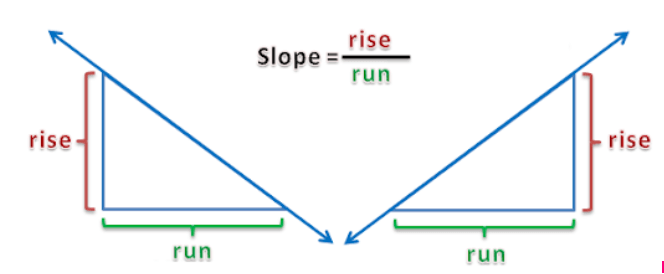# Finding the Gradient of a Straight Line

July 24, 2020
SAC TutoringGradient? Isn’t that like a colour thing?

In maths the gradient has a slightly different meaning.

The gradient of a straight line shows us how steep the line is. Think of the mountain side.

In worded form, the gradient is rise over run.

There are two types of gradients; negative and positive.

Negative gradients go from up to down. Like when you drop your ice cream, your mood goes from up to down. Now you’re feeling negative :(

Positive gradients go from down to up. Like when you get another ice cream your mood goes up and you’re back to feeling positive :)

## Finding the Gradient in a Linear Equation

A linear equation shows the equation of a straight line.

Here we can see that m = gradient and c = y intercept.

Note: the y intercept is the point on the y axis where the line crosses.

So, if we have the equation of a straight line, we can simply find m and there’s our gradient.

Example 1: Find the gradient of this equation.

We can see that this equation has the same format as our linear equation.

This means that ½ is our gradient. So, to answer the question, the gradient of this line is ½.

Now what if our equation doesn’t have the same format as our linear equation?

Don’t stress! All we have to do is rearrange it a bit by transposing!

Example 2:

Here, our equation is not in the same format as the linear equation. Let’s start moving things around to try get that y by itself.

First, we’re going to move the x to the other side of the equals sign.

Note: x is positive so to move it over, we change the sign to a negative.

This gives us

Now, to get y by itself, we need to move that 3.

Note: the 3 is multiplied so to move it over, we need to divide.

This give us

Next, we simplify.

Okay, but wait, which one is m?

Well, it's the number in front of the x so in this case it would be -⅓.

## Finding the Gradient Using 2 Points

For these types of question we need to use a formula

The x and y just refer to the points given in the question.

So, x1 is the x coordinate of the first point and y1 is the y coordinate of the first point. x2 is the x coordinate of the second point and y2 is the second coordinate of the second point.

Let’s look at an example.

Example 1

Step 1: Label the coordinates.

Step 3: Plug in your coordinates into the formula.

Step 4: As always, we have to simplify!